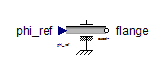Derivative - MapleSim Help

Derivative

Compute an approximate derivativeDescription The Derivative component computes an element-wise approximate derivative. Each element has transfer function $\frac{{y}_{j}\left(s\right)}{{u}_{j}\left(s\right)}=\frac{ks}{Ts+1}$. The Signal Size parameter assigns the dimension of the input and output connectors.
 Equations $\stackrel{.}{{x}_{j}}=\left\{\begin{array}{cc}0& \mathrm{zeroGain}\\ \frac{{u}_{j}-{x}_{j}}{T}& \mathrm{otherwise}\end{array}$ ${y}_{j}=\left\{\begin{array}{cc}0& \mathrm{zeroGain}\\ \frac{k}{T}\left({u}_{j}-{x}_{j}\right)& \mathrm{otherwise}\end{array}$ $\mathrm{zeroGain}=\left(\left|k\right|<\mathrm{\epsilon }\right)$

Connections

 Name Description Modelica ID $u$ Real input vector u $y$ Real output vector y

Parameters

 Name Default Units Description Modelica ID k $1$ Gain k T $0.01$ $s$ Time constant.  Must be positive T Initial Values No initialization Type of initialization. initType ${x}_{0}$ $0$ Initial or guess value of state x_start ${y}_{0}$ $0$ Initial value of output y_start Signal Size $1$ Dimension of input and output signals signalSize

 Modelica Standard Library The component described in this topic is from the Modelica Standard Library. To view the original documentation, which includes author and copyright information, click here.